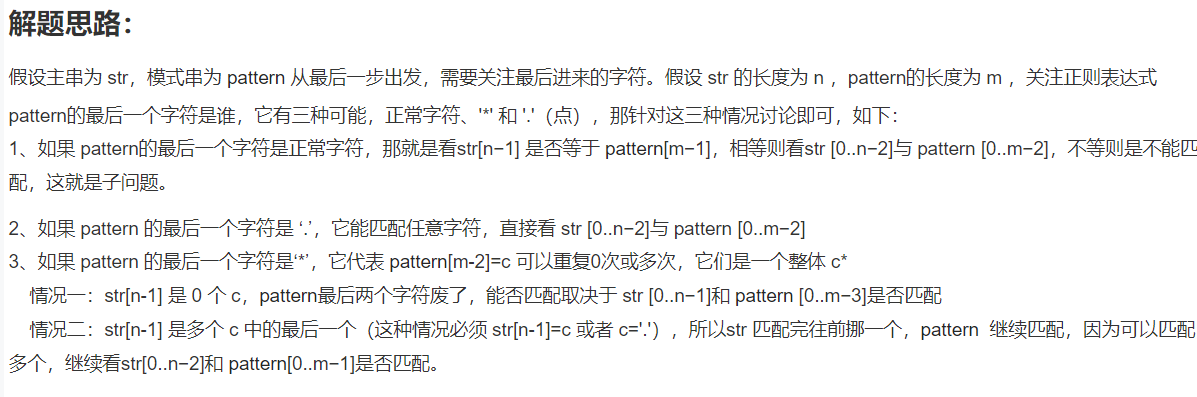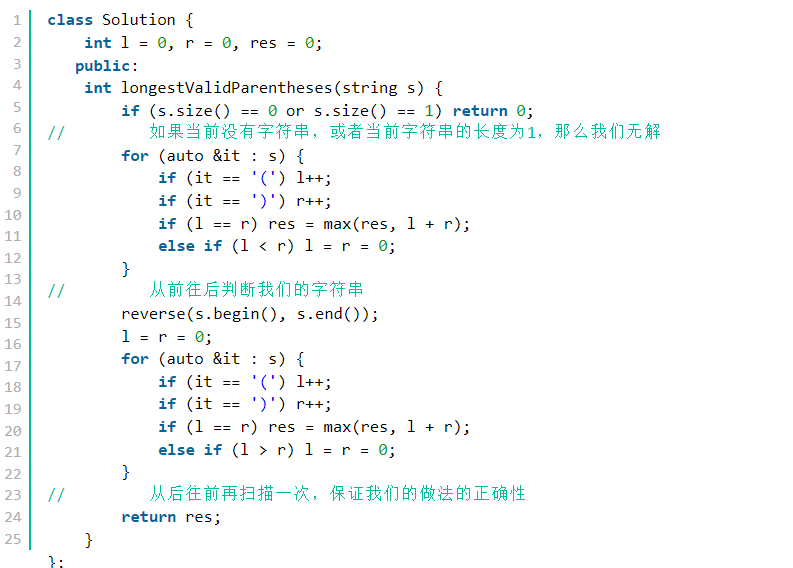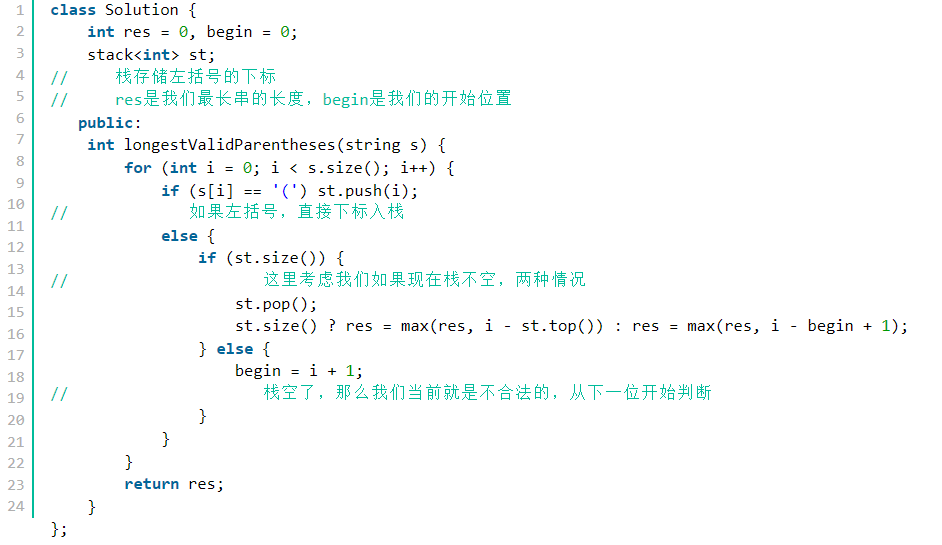﻿• 价格透明
• 信息保密
• 进度掌控
• 售后无忧# 按照方法分类

## （三）动态规划问题（cn.）

### 13、 给定一个长度为 n 的数组 arr，求它的最长严格上升子序列的长度。

• 子序列：指一个数组删掉一些数（也可以不删）之后，形成的新数组。
• 严格上升 ：该序列不存在两个下标 i 和 jj满足 i<j 且 arr[i]≥arr[j]。
• 动态规划，dp[i]表示以原数组中的数arr[i]结尾的最长严格上升子序列的长度。
• dp[i]初始值均为1；
• 状态转移方程：dp[i]=max(dp[i],dp[j]+1)
• 对于每个数arr[i]，依次遍历它前面的数字arr[j] (i<j)。
• 如果前面的数字小，说明以arr[j]结尾的最长严格子序列加上arr[i]之后仍然是严格上升的（新的子序列记为a），此时以arr[i]结尾的最长严格上升子序列的长度可能得到更新，当然也可能不变（a的长度小于等于dp[i]）

### 14、 连续子数组的最大和

• dp[i]表示以原数组中的第i个数arr[i]结尾的连续数组的最大和。
• dp[i]初始值为本身；
• 状态转移方程：dp[i]=max(dp[i],dp[i-1]+i)

### 15、 最长回文子串

• 维护一个布尔型的二维数组dp，dp[i][j]表示 i 到 j 的子串是否是回文子串。 每次先判断边界字符是否相等，再取决于上个状态的判断结果；
• (1) `dp[i][j]=dp[i+1][j-1]&&(A[i]==A[j]) if j>i+1`（判断字符串首尾两字符相等，且去掉首尾两字符后的字符串为回文串，便可得当前字符串为回文串）
• (2) `dp[i][j]=(A[i]==A[j]) if j==i+1`（字符串长度为1的情况）
• (3) `dp[i][j]=1 if j==i`（字符串长度为1和2的情况）
• 中心向外扩展法，每个值都要成为中心，分二中心和一中心，需要同时比较。

• 回溯

### 17、 给定两个字符串 str1 和 str2 ，请你算出将 str1 转为 str2 的最少操作数

• 三种情况——添加、插入、删除
• DFS
• vis[i][j]代表的就是我们到了str1的第i位str2的第j位
• 动态规划
• dp[i][j]表示为从str1的第i位变化位str2的第j位的次数
• str1第i位和str2第j位一样 `dp[i][j]=dp[i−1][j−1]`
• str1第i位和str2第j位不一样 `dp[i][j]=min(dp[i−1][j−1],min(dp[i−1][j],dp[i][j−1]))+1`//对应三种情况

### 18、 实现一个函数用来匹配包括’.‘和’*'的正则表达式

#### （1） 初始条件：• 空串和空正则是匹配的，f=true；
• 非空串和空正则必不匹配，f=…=f[n]=false；
• 空串和非空正则，不能直接定义 true 和 false，必须要计算出来。（比如str= ‘’ ‘’ ,pattern=abc*）； 非空串和非空正则，那肯定是需要计算的了。

#### （2） 转移方程：

• f[i][j] 代表 str 的前 i 个和 pattern 的前 j 个能否匹配
• 对于前面两个情况，可以合并成一种情况 f[i][j]=f[i−1][j−1]
• 对于第三种情况，对于 c* 分为看和不看两种情况
• 不看：直接砍掉正则串pattern 的后面两个， f[i][j]=f[i][j−2]
• 看：正则串pattern 不动，主串str前移一个，f[i][j]=f[i−1][j]

#### （3） 结果：

• 数组大小为[n+1 ,m+1]，这样对于空串的处理十分方便。
• 结果就是 f[n][m]

### 19、 给出一个长度为 n 的，仅包含字符 ‘(’ 和 ‘)’ 的字符串，计算最长的格式正确的括号子串的长度

#### （1）方法1： 动态规划（好难）

• dp[i] 表示以下标 i 字符结尾的最长有效括号的长度。将 dp 数组全部初始化为 0 。显然有效的子串一定以 ‘)’ 结尾，因此我们可以知道以 ‘(’ 结尾的子串对应的 dp 值必定为 0 ，我们只需要求解 ‘)’ 在 dp 数组中对应位置的值
• s[i] = ‘)’ 且 s[i-1] = ‘(’ dp[i] = dp[i-2] + 2
• s[i] = ‘)’ 且 s[i-1] = ‘)’ 如果s[i - dp[i-1] - 1] = ‘(’，则 dp[i] = dp[i-1] + dp[i-dp[i-1]-2] + 2

#### （2）方法2： 贪心

• 从前向后看的话, 我们可以确定必须严格保证时刻左括号的数量时刻大于等于右括号数量，如果不满足, 就把左右指针同时清零
• 反向遍历的时候,要严格保证右括号比左括号多，如果不满足, 就把左右指针同时清零#### （3）方法3： 栈

• 用一个变量维护合法子串的起始位置, 然后如果是左括号直接把下标入栈, 如果是右括号弹出栈顶元素, 然后更新答案
• 这里有两种情况：如果栈空了, 那么res为当前位置到我们的起始位置；如果栈不空, 就是当前位置到前一个左括号的位置### 低价透明### 金牌服务### 信息保密